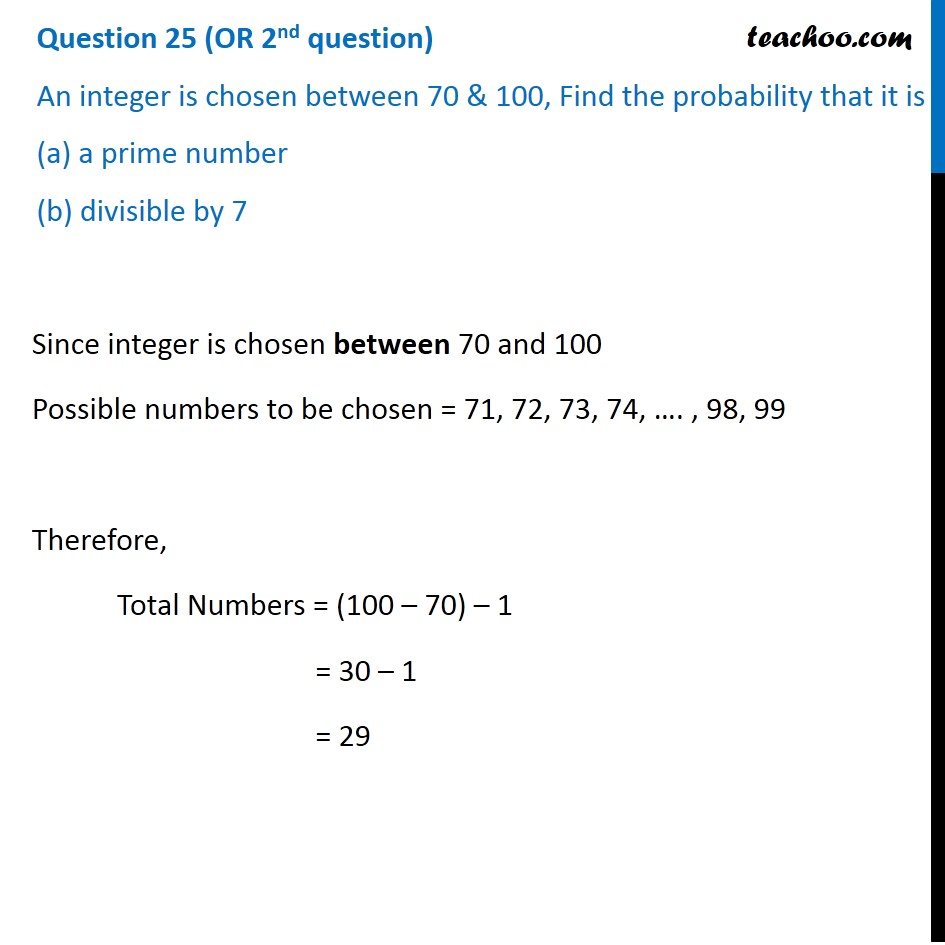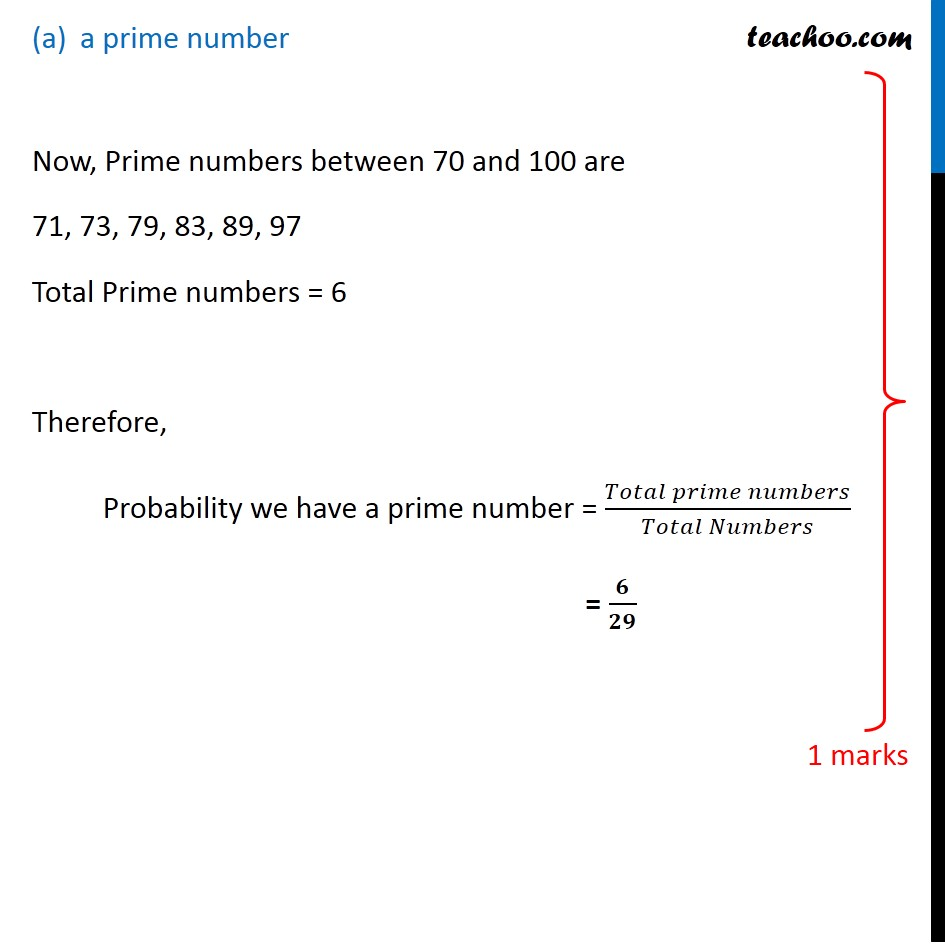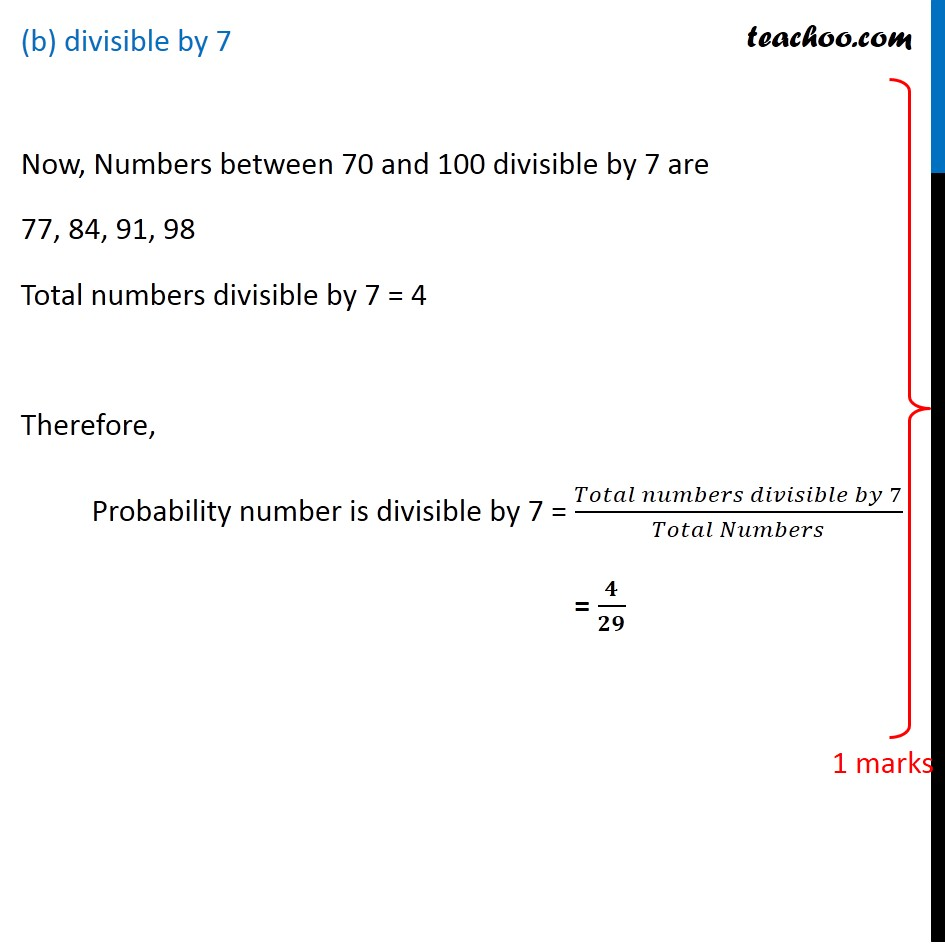CBSE Class 10 Sample Paper for 2020 Boards - Maths Standard

Class 10
Solutions of Sample Papers for Class 10 Boards

## (b) divisible by 7Note : To see Prime numbers from 1 to 100 , please check

Learn in your speed, with individual attention - Teachoo Maths 1-on-1 Class

### Transcript

Question 25 (OR 2nd question) An integer is chosen between 70 & 100, Find the probability that it is (a) a prime number (b) divisible by 7 Since integer is chosen between 70 and 100 Possible numbers to be chosen = 71, 72, 73, 74, …. , 98, 99 Therefore, Total Numbers = (100 – 70) – 1 = 30 – 1 = 29 a prime number Now, Prime numbers between 70 and 100 are 71, 73, 79, 83, 89, 97 Total Prime numbers = 6 Therefore, Probability we have a prime number = (𝑇𝑜𝑡𝑎𝑙 𝑝𝑟𝑖𝑚𝑒 𝑛𝑢𝑚𝑏𝑒𝑟𝑠)/(𝑇𝑜𝑡𝑎𝑙 𝑁𝑢𝑚𝑏𝑒𝑟𝑠) = 𝟔/𝟐𝟗 (b) divisible by 7 Now, Numbers between 70 and 100 divisible by 7 are 77, 84, 91, 98 Total numbers divisible by 7 = 4 Therefore, Probability number is divisible by 7 = (𝑇𝑜𝑡𝑎𝑙 𝑛𝑢𝑚𝑏𝑒𝑟𝑠 𝑑𝑖𝑣𝑖𝑠𝑖𝑏𝑙𝑒 𝑏𝑦 7)/(𝑇𝑜𝑡𝑎𝑙 𝑁𝑢𝑚𝑏𝑒𝑟𝑠) = 𝟒/𝟐𝟗